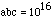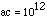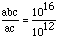Date: Mon, 27 Jul 1998 17:27:19 -0600 (CST)
Subject: Trig

Name: James

Level: Secondary

Question:
How do you solve these problem? If log abc=16 and log ac=12 , find b. (The logs are log base 10.)
and
If a and b are real numbers, i^2 = -1 and (a+b)+5i=9+ai what is the value of b?

Hi again James
For the log problem remember that logarithms can be written as exponents. Your equations can be re-written as:andIf we divided equation 1 by equation 2 we obtain:hence b = 104.

For your second problem, if x, y, s, and t are real numbers then the complex numbers x + yi and s + ti are equal if and only if x = s and y = t. So a + b = 9 and 5 = a. Thus a = 5 and b = 4.

Good luck,
Jack

Go to Math Central